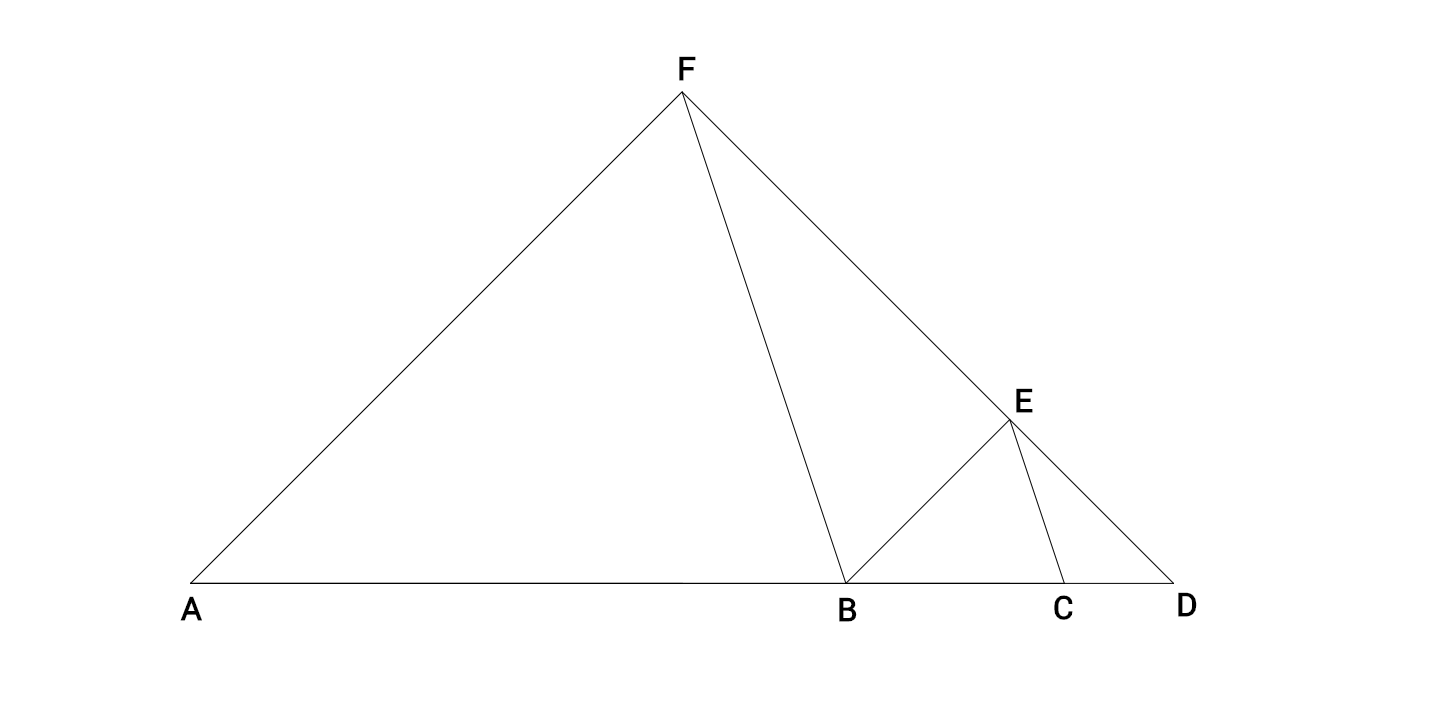# Triangle-Ception III

Geometry Level 4In the figure above, $\triangle ABF \sim \triangle BCE$, with $\angle FAB = \angle EBC$ and $\angle AFB = \angle BEC=109^{\circ}$.

Additionally, points $A,B,C,D$ are collinear and points $D,E,F$ are collinear.

Given that $AC=8$ and $BD=6$, the length of $AD$ can be expressed in the form $m+n\sqrt{p}$ where $m,n,p$ are positive integers, and $p$ is not divisible by the square of any prime.

Find $m+n+p$.

×

Problem Loading...

Note Loading...

Set Loading...